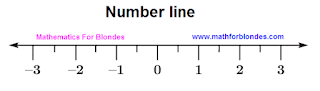## 2/08/2011

### Division by zero - the question formulation

Division by zero is maybe - to such conclusion we have come. But to solve a problem about division of number into a zero we and could not. Then let's solve not a specific target on division of number into a zero, and a zero problem as a whole. We begin all from the very beginning.

What is the zero? All-knowing Wikipedia says that the zero is a number. This number designates a point on a numerical straight line which separates positive numbers from the negative. Give also we will look at this well-known numerical straight line in which the dog on a nickname "Zero" is buried.Number line

And now we will look, as the zero in the basic mathematical operations behaves agrees the standard mathematical rules:

a + 0 = a

0 + a = a

a - 0 = a

0 - a = -a

a - a = 0

a · 0 = 0

a : 0 = ?

0 : a = 0

0 : 0 = ?

In that mathematicians are mistaken, including division into a zero impossible, we have already understood. Instead of whether and mathematicians are mistaken in other places at the formulation of results of mathematical actions with zero? Quite probably that some from resulted above equalities are false statements.

The problem with zero in the mathematician dares simply and gracefully, in style of blondes. Therefore mathematicians to such never will guess. Here the sober and critical sight of the person from outside, without fanatical belief in the received mathematical knowledge is necessary. Blondes for a zero solution of a problem approach as well as possible. About the mathematician they have the most general representations. Their mentality differs from the standard.

Whether you can find a solution of a problem with zero? The variants of the decision leave in comments.